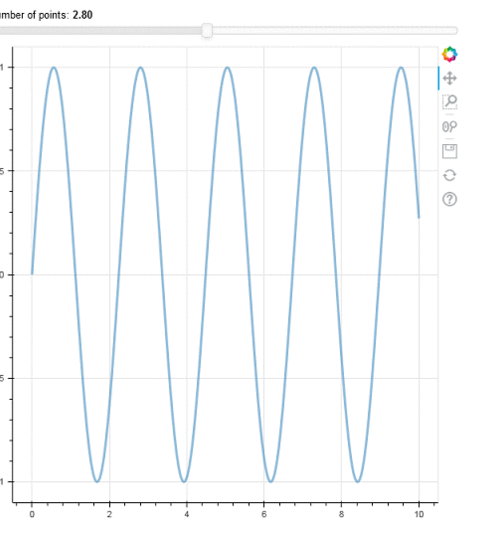# Add Interactive Slider to Bokeh Plots

• Last Updated : 28 Jul, 2021

Bokeh is an interactive Data visualization library of Python. It can be used to create interactive plots, dashboards, and data applications. Widgets are nothing but additional visual elements that you can add to your plots to interactively control your Bokeh document. There are various types of widgets such as button, div, spinner, slider, etc. In this article, we will learn about the slider widget in bokeh.

## Slider Widget

The Bokeh slider can be configured with start and end values, a step size, an initial value, and a title. Basically, you need to import the Slider widget from bokeh.models.

Attention geek! Strengthen your foundations with the Python Programming Foundation Course and learn the basics.

To begin with, your interview preparations Enhance your Data Structures concepts with the Python DS Course. And to begin with your Machine Learning Journey, join the Machine Learning - Basic Level Course

Syntax:

from bokeh.models import CustomJS, Slider

Slider() function can be used to create a slider.

Syntax:

Slider(start=0, end=10, value=1, step=.1, title=”Stuff”)

Parameter:

• start: It represents the sliders starting value.
• end:It represents the sliders ending value.
• value: It represents the current value of the slider.
• step: It represents the interval through which the slider moves.
• title: It represents the title of the slider widget.

Now add callback functionality using CustomJS which is called when on_change event occurs.

Syntax:

js_on_change(“value”, CustomJS(code=”””…. “””))

js_on_change is a callback function that is called when slider on_change event occurs. and customJS(code=”””   “””) represents the code that is to be executed once the event occurs. Now call the callback function using the slider object and create a layout of all the elements you want to display on the browser.

Example: Creating a slider using bokeh

## Python

 `from` `bokeh.layouts ``import` `column``from` `bokeh.models ``import` `ColumnDataSource, Slider, CustomJS``from` `bokeh.plotting ``import` `figure, output_file, show``import` `numpy as np`` ` `x ``=` `np.linspace(``0``, ``10``, ``500``)``y ``=` `np.sin(x)`` ` `source ``=` `ColumnDataSource(data``=``dict``(x``=``x, y``=``y))`` ` `# Create plots and widgets``plot ``=` `figure()`` ` `plot.line(``'x'``, ``'y'``, source``=``source, line_width``=``3``, line_alpha``=``0.5``)`` ` `# Create Slider object``slider ``=` `Slider(start``=``0``, end``=``6``, value``=``2``,``                ``step``=``0.2``, title``=``'Number of points'``)`` ` `# Adding callback code``callback ``=` `CustomJS(args``=``dict``(source``=``source, val``=``slider),``                    ``code``=``"""``    ``const data = source.data;``    ``const freq = val.value;``    ``const x = data['x'];``    ``const y = data['y'];``   ``for (var i = 0; i < x.length; i++) {``        ``y[i] = Math.sin(freq*x[i]);``    ``}``     ` `    ``source.change.emit();``"""``)`` ` `slider.js_on_change(``'value'``, callback)`` ` `# Arrange plots and widgets in layouts``layout ``=` `column(slider, plot)`` ` `output_file(``'exam.html'``)`` ` `show(layout)`

Output:My Personal Notes arrow_drop_up